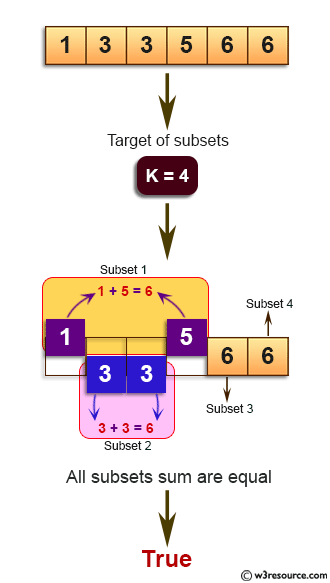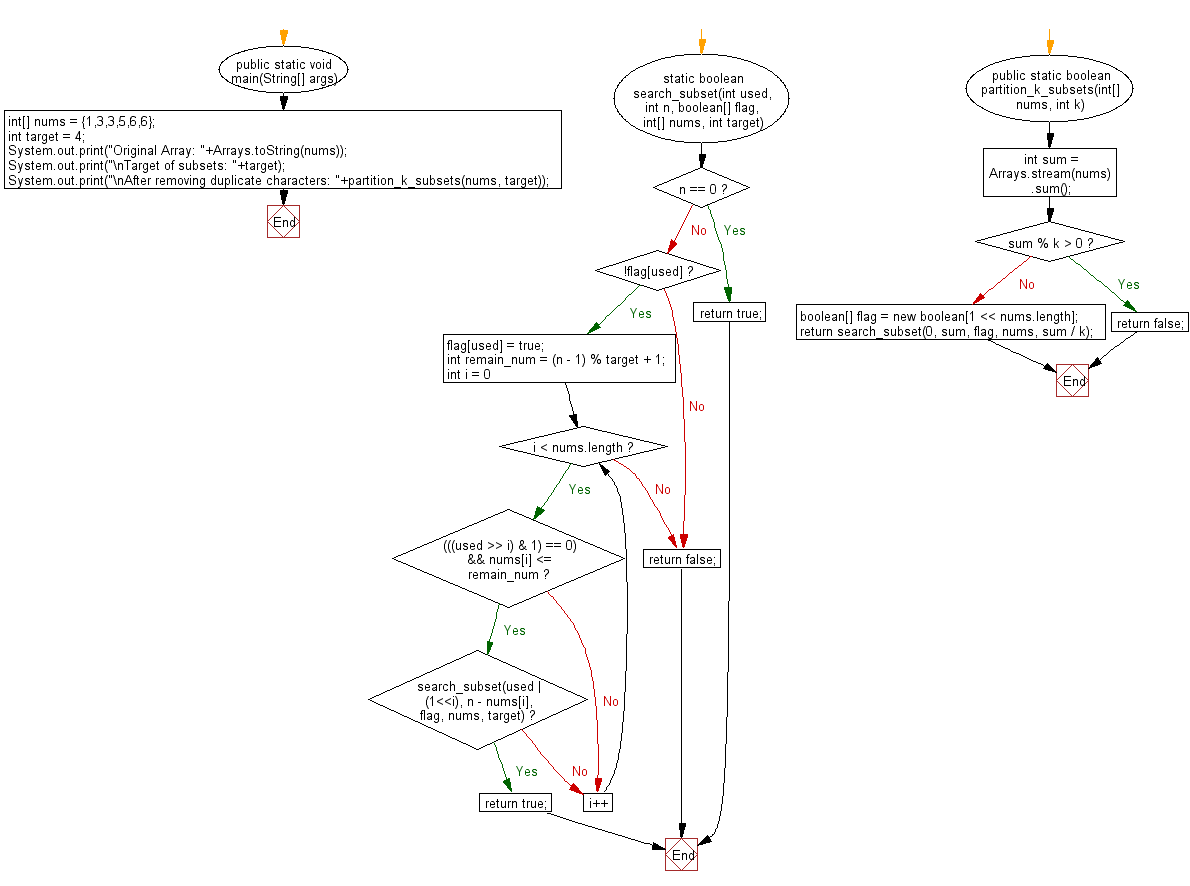# Java Exercises: Divide a given array of integers into given k non-empty subsets whose sums are all equal

## Java Basic: Exercise-201 with Solution

Write a Java program to divide a given array of integers into given k non-empty subsets whose sums are all equal. Return true if all sums are equal otherwise return false.

Example:
nums = {1,3,3,5,6,6}, k = 4;
4 subsets (5,1), (3, 3), (6), (6) with equal sums.

Pictorial Presentation:Sample Solution:

Java Code:

``````import java.util.*;

public class Solution {
public static void main(String[] args) {
int[] nums = {1,3,3,5,6,6};
int target = 4;
System.out.print("Original Array: " + Arrays.toString(nums));
System.out.print("\nTarget of subsets: " + target);
System.out.print("\nAfter removing duplicate characters: " + partition_k_subsets(nums, target));
}
static boolean search_subset(int used, int n, boolean[] flag, int[] nums, int target) {
if (n == 0) {
return true;
}

if (!flag[used]) {
flag[used] = true;
int remain_num = (n - 1) % target + 1;
for (int i = 0; i & lt; nums.length; i++) {
if ((((used >> i) & 1) == 0) && nums[i] & lt; = remain_num) {
if (search_subset(used | (1 & lt; & lt; i), n - nums[i], flag, nums, target)) {
return true;
}
}
}
}
return false;
}

public static boolean partition_k_subsets(int[] nums, int k) {
int sum = Arrays.stream(nums).sum();
if (sum % k > 0) {
return false;
}
boolean[] flag = new boolean[1 & lt; & lt; nums.length];
return search_subset(0, sum, flag, nums, sum / k);
}
}
```
```

Sample Output:

```Original Array: [1, 3, 3, 5, 6, 6]
Target of subsets: 4
After removing duplicate characters: true
```

Flowchart:Java Code Editor:

Company:

What is the difficulty level of this exercise?

﻿

## Java: Tips of the Day

Parsing dates:

```import java.io.*;
import java.util.*;
import java.text.*;

String s = "2001/09/23 14:39";

SimpleDateFormat formatter = new SimpleDateFormat ("yyyy/MM/dd H:mm");
Date d = formatter.parse(s, new ParsePosition(0));
```